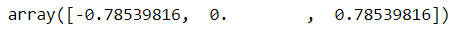Prerequisite: Array Arithmetic Operations in NumPy

Apart from arithmetic operations, NumPy also gives us some very useful Trigonometric functions used in Data Analysis.

#### Trigonometric sin(), cos(), tan() functions in NumPy

Code

```import numpy as np
pi = np.pi
np.sin(pi)
```

Output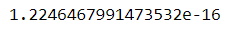Code

```np.cos(pi)
```

Output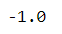Code

```np.tan(pi)
```

Output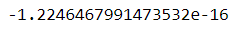Note: The values are computed to within machine precision, which is why values that should be zero do not always hit exactly zero.

#### sin(), cos() & tan() in NumPy Array

The Trigonometric sin(), cos() & tan() functions work exactly the same for NumPy array as they work for a single constant value.

Code

```theta = np.array([0, pi/2, 3*pi/2, 2*pi])
np.sin(theta)
```

Output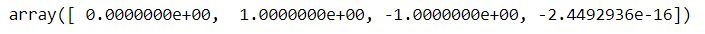Code

```np.cos(theta)
```

Output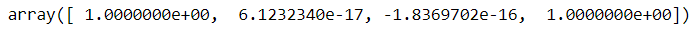Code

```np.tan(theta)
```

Output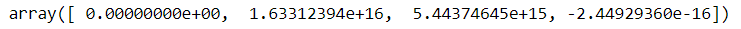#### Inverse Trigonometric Functions

The arcsin(), arccos() & arctan() functions in NumPy work as inverse functions for sin(), cos() & tan() respectively.

Code

```x = np.array([-1, 0, 1])
np.arcsin(x)
```

Output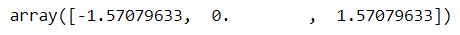Code

`np.arccos(x) `

Output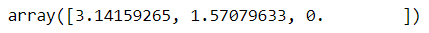Code

```np.arctan(x)
```

Output